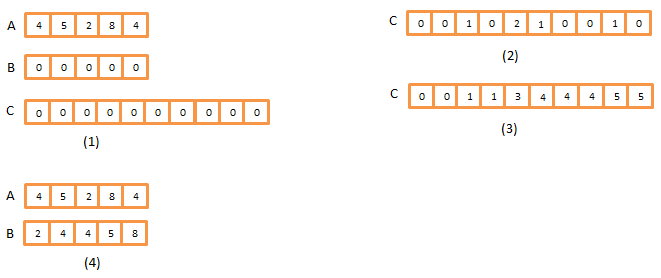# Implementation of Counting Sort with C# and analysis of running time

 Category: Data Structure And Algorithms Tags:Counting Sort Code Files

## Introduction

Previously we have analyzed almost five algorithms(insertion, merge, heap, quick, selection) which all are well known algorithms. All of these algorithms work on comparisons where algorithm compares elements to other elements and find the exact position of elements, and runs on average running time between [n2] and [n log n] but counting sort doesn't compare elements and runs on linear time [n]. No other comparison sort take less than n log n time but here we are going to sort an array in linear time.

As name describes, counting sort counts how many elements are actually smaller than an element and using this observation it re-position the elements in new output array.

## Implementation

Implementation of counting sort is given below

```public class CountingSort
{
public int lengthTemp = 100;
public int indexMaxFilled = 0;
public CountingSort(int[] array)
{
A = array;
}
public int[] Sort()
{
int[] B = new int[A.Length];
int[] C = new int[lengthTemp];
for (int i = 0; i < A.Length; i++)
{
C[A[i]] = C[A[i]] + 1;
if (indexMaxFilled < A[i])
indexMaxFilled = A[i];
}
for (int i = 1; i <= indexMaxFilled; i++)
{
C[i] = C[i] + C[i - 1];
}
for (int j = A.Length - 1; j >= 0; j--)
{
B[C[A[j]] - 1] = A[j];
C[A[j]] = C[A[j]] - 1;
}
return B;
}
}```

We can see A is the array which needs to be sorted and inside method Sort we have two arrays B and C where B is same length as A will be used to store output and C is a temporary array. There is a strange thing about C is we have a temporary length which should be equal or grater than largest element of input array, for example we have elements from 1 to 300 in array A than array C should have last index 300 or bigger, means you cannot have 299 as last index if largest element is 300 in array. But we may modify this algorithm about this limitation also which I will not cover in this article.

So, inside method Sort we have 3 loops, in first loop we assume the Array index of C as numbers to be sorted and we set count how many times it is getting repeated. Suppose we have input A{4,5,2,8,4} and let's suppose C{0,0,0,0,0,0,0,0,0,0} which is initialized by length 10 and is greater than the largest number of A (last index of C is 9 > largest number of A is 8). Now after first loop the output will come as {0,0,1,0,2,1,0,0,1,0} means number 0 and 1 is not in input so index 0 and index 1 of C is still 0 but number 2 appeared once in input so index 2 of C is flagged by 1.

The second loop is to calculate to every number in Array C, like how many are smaller or equal inputs are available than a number, so after second loop the array C will be {0,0,1,1,3,4,4,4,5,5} means index zero of C which we assume as number(number which might be in input to sort) is no elements less or equal than it, index 1 is also flagged 0, but index 2 is flagged with 1 means we have one number less or equal to it and so on, simply we will be adding a index value with its previous index value to get the count. So here we have everything cooked now only needs to server it

The third loop does the serving work, here we run a loop number of time as input length and finds the correct position of each element in A and place it in B. See figure below:In All the figures 1-4 we can see the main role of Array C which keeps counts of how many smaller elements are present than any element, in array C we take index value as element. Let's run above code:

`CountingSort countingSort = new CountingSort(new int[] { 2, 1, 7, 4, 9, 3, 6 });var sortedArr = countingSort.Sort();foreach (int ele in sortedArr)    Console.WriteLine(ele);Console.ReadLine();`

Output

1

2

3

4

6

7

9

## Analysis

It is easy to figure out the running time here we have 3 loops where first will take time Θ(n) the second takes Θ(k) (k is some number equals to indexMaxFilled property in code) and third one takes Θ(n) so running time is Θ(2n + k) so it shows a linear time Θ(n). So Counting sort runs on linear time which beats running time of quick sort(n log n).

 Like1 PersonNikhil Joshi Ceo & Founder at Dotnetlovers Atricles: 135 Questions: 9 Given Best Solutions: 9 *

Login via:x